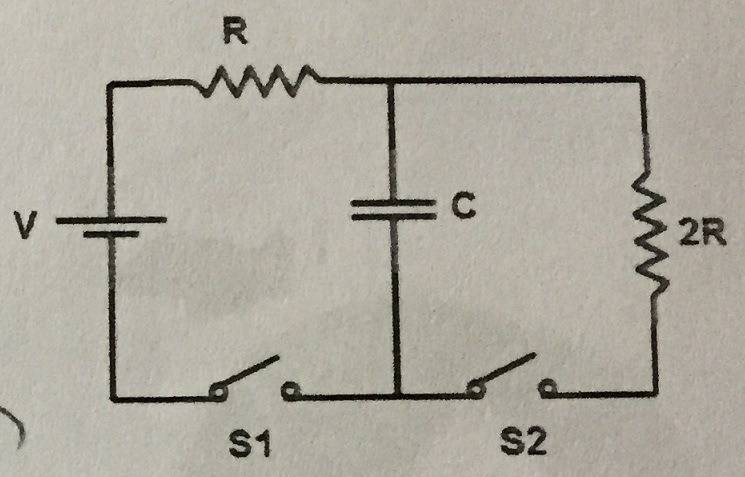# RC Circuit Help

## Homework Statement

There is a circuit with a battery of voltage V and resistor R, then splits into two branches one with a capacitor C, and one with a resistor 2R.

The answers for the questions can only depend on R, C, V, and t.

When a switch only allows current to flow through the capacitor branch, the expression for charge is:

##Q = C V (1-e^{-t/(RC)})##

a) When the switch is closed, find an expression for the charge on the capacitor after a very long time (t >> RC)

b) When the switch is closed, find an expression for the current through the battery after a very long time (t >> RC)

## Homework Equations

##Q = C V (1-e^{-t/(RC)})##

Current through resistor R:
##I = (V / R) e^{-t/(RC)}##

Current through resistor 2R:
##I = (-V / 2R) e^{-t/(2RC)}##

## The Attempt at a Solution

a) I tried taking the limit of the charge as t goes to infinity and got my answer to be CV, but I only received 1/4 possible points for the problem so I either didn't do it right or didn't show all the work.

b) I tried taking the limit of the current and t goes to infinity and got my answer to be 0, but that is also wrong.

NascentOxygen
Staff Emeritus
Hi EROC. http://img96.imageshack.us/img96/5725/red5e5etimes5e5e45e5e25.gif [Broken]

1) A good diagram is essential! It helps you explain what you are talking about, helps those who respond, and helps other students understand what is going on.

2) For the voltage at any point as t → ∞, consider what it would be if the capacitor were not in the crcuit. That's what it will settle at when the capacitor is present, too.

Last edited by a moderator:
To rephrase the questions with the actual diagram (because I didn't know how to upload it with the diagram because it said no attachments)a)When S1 and S2 are closed, find an expression for the charge on the capacitor after a very long time (t >> RC)

2) For the voltage at any point as t → ∞, consider what it would be if the capacitor were not in the crcuit. That's what it will settle at when the capacitor is present, too.

So there would be the voltage of V-IR on the capacitor but what would be the charge, would I just have to solve for ##Q=C(V-IR)## and then solve for the current when acting like the capacitor is not in the circuit.

b)When S1 and S2 are closed, find an expression for the current through the battery after a very long time (t >> RC)

NascentOxygen
Staff Emeritus